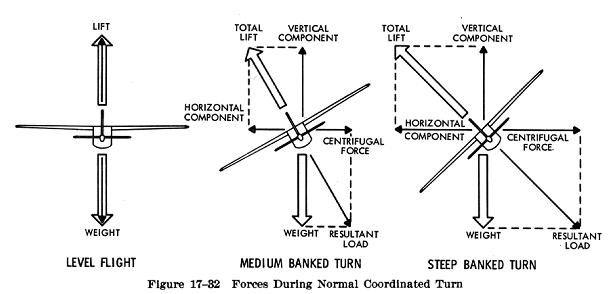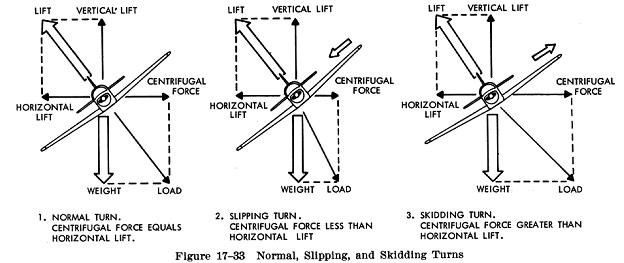Forces in Turns Forces in Turns

If an airplane were viewed in straight and level flight from the rear (Fig. 17-32), and if the forces acting on the airplane actually could be seen, two forces (lift and weight) would be apparent, and if the airplane were in a bank it would be apparent that lift did not act directly opposite to the weight - it now acts in the direction of the bank. The fact that when the airplane banks, lift acts inward toward the center of the turn, as well as upward, is one of the basic truths to remember in the consideration of turns.As we learned earlier, an object at rest or moving in a straight line will remain at rest or continue to move in a straight line until acted on by some other force. An airplane, like any moving object, requires a sideward force to make it turn. In a normal turn, this force is supplied by banking the airplane so that lift is exerted inward as well as upward. The force of lift during a turn is separated into two components at right angles to each other. One component which acts vertically and opposite to the weight (gravity) is called the "vertical component of lift." The other which acts horizontally toward the center of the turn is called the "horizontal component of lift." The horizontal component of lift is the force that pulls the airplane from a straight flightpath to make it turn. Centrifugal force is the "equal and opposite reaction" of the airplane to the change in direction and acts equal and opposite to the horizontal component of lift. This explains why, in a correctly executed turn, the force that turns the airplane is not supplied by the rudder.

An airplane is not steered like a boat or an automobile; in order for it to turn, it must be banked. If the airplane is not banked, there is no force available that will cause it to deviate from a straight flightpath. Conversely, when an airplane is banked, it will turn, provided it is not slipping to the inside of the turn. Good directional control is based on the fact that the airplane will attempt to turn whenever it is banked. This fact should be borne in mind at all times, particularly while attempting to hold the airplane in straight and level flight.

Merely banking the airplane into a turn produces no change in the total amount of lift developed. However, as was pointed out, the lift during the bank is divided into two components, one vertical and the other horizontal. This division reduces the amount of lift which is opposing gravity and actually supporting the airplane's weight; consequently, the airplane loses altitude unless additional lift is created. This is done by increasing the angle of attack until the vertical component of lift is again equal to the weight. Since the vertical component of lift decreases as the bank angle increases, the angle of attack must be progressively increased to produce sufficient vertical lift to support the airplane's weight. The fact that the vertical component of lift must be equal to the weight to maintain altitude is an important fact to remember when making constant altitude turns.

At a given airspeed, the rate at which an airplane turns depends upon the magnitude of the horizontal component of lift. It will be found that the horizontal component of lift is proportional to the angle of bank; that is, it increases or decreases respectively as the angle of bank increases or decreases. It logically follows then, that as the angle of bank is increased the horizontal component of lift increases, thereby increasing the rate of turn. Consequently, at any given airspeed the rate of turn can be controlled by adjusting the angle of bank.

To provide a vertical component of lift sufficient to hold altitude in a level turn, an increase in the angle of attack is required. Since the drag of the airfoil is directly proportional to its angle of attack, induced drag will increase as the lift is increased. This, in turn, causes a loss of airspeed in proportion to the angle of bank; a small angle of bank results in a small reduction in airspeed and a large angle of bank results in a large reduction in airspeed. Additional thrust (power) must be applied to prevent a reduction in airspeed in level turns; the required amount of additional thrust is proportional to the angle of bank.

To compensate for added lift which would result if the airspeed were increased during a turn, the angle of attack must be decreased, or the angle of bank increased, if a constant altitude were to be maintained. If the angle of bank were held constant and the angle of attack decreased, the rate of turn would decrease. Therefore, in order to maintain a constant rate of turn as the airspeed is increased, the angle of attack must remain constant and the angle of bank increased.

It must be remembered that an increase in airspeed results in an increase of the turn radius and that centrifugal force is directly proportional to the radius of the turn. In a correctly executed turn, the horizontal component of lift must be exactly equal and opposite to the centrifugal force. Therefore, as the airspeed is increased in a constant rate level turn, the radius of the turn increases. This increase in the radius of turn causes an increase in the centrifugal force, which must be balanced by an increase in the horizontal component of lift can only be increased by increasing the angle of bank.

In a slipping turn the airplane is not turning at the rate appropriate to the bank being used, since the airplane is yawed toward the outside of the turning flightpath. The airplane is banked too much for the rate of turn, so the horizontal lift component is greater than the centrifugal force (Fig. 17-33). Equilibrium between the horizontal lift component and centrifugal force is reestablished either by decreasing the bank, increasing the rate of turn, or a combination of the two changes.A skidding turn results from an excess of centrifugal force over the horizontal lift component, pulling the airplane toward the outside of the turn. The rate of turn is too great for the angle of bank. Correction of a skidding turn thus involves a reduction in the rate of turn, an increase in bank, or a combination of the two changes.

To maintain a given rate of turn, the angle of bank must be varied with the airspeed. This becomes particularly important in high speed airplanes. For instance, at 400 miles per hour, an airplane must be banked approximately 44 degrees to execute a standard rate turn (3 degrees per second). At this angle of bank, only about 79 percent of the lift of the airplane comprises the vertical component of the lift; the result is a loss of altitude unless the angle of attack is increased sufficiently to compensate for the loss of vertical lift.